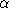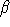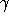## CGAL::Arr_circle_segment_traits_2<Kernel>

### Definition

The class Arr_circle_segment_traits_2<Kernel> is a model of the ArrangementTraits_2 concept and can be used to construct and maintain arrangements of circular arcs and line segments.

The traits class must be instantiated with a geometric kernel, such that the supporting circles of the circular arcs are of type Kernel::Circle_2 and the supporting lines of the line segments are of type Kernel::Line_2. Thus, the coordinates of the center of supporting circles, and its squared radius are of type Kernel::FT, which should be an exact rational number-type; similarly, the coefficients of each supporting line ax + by + c = 0 are also of type Kernel::FT. Note however that the intersection point between two such arcs do not have rational coordinates in general. For this reason, we do not require the endpoints of the input arcs and segments to have rational coordinates.

The nested Point_2 type defined by the traits class is therefore different than the Kernel::Point_2 type. Its coordinates are of type CoordNT, and represent real numbers obtained from solving quadratic equations with rational coordinates. A number of type CoordNT can therefore be expressed as+sqrt(), where,andare all rational numbers. The definition of the curve and x-monotone curve types nested in the traits class are detailed below.

#include <CGAL/Arr_circle_segment_traits_2.h>

### Is Model for the Concepts

ArrangementTraits_2

### Class Arr_circle_segment_traits_2<Kernel>::CoordNT

The CoordNT number-type nested within the traits class represents an algebraic number of degree 2; it can be represented as+sqrt(), where,andare all rational numbers of type Kernel::FT.

### Types

 Arr_circle_segment_traits_2::CoordNT::Rational the Kernel::FT type.

### Creation

 Arr_circle_segment_traits_2::CoordNT x; creates a variable whose value is 0. Arr_circle_segment_traits_2::CoordNT x ( Rational alpha); creates a variable whose value is the rational number. Arr_circle_segment_traits_2::CoordNT x ( Rational alpha, Rational beta, Rational gamma); creates a variable whose value is the algebraic number+sqrt().

### Access Functions

 bool x.is_rational () determines whether x is rational. Rational x.alpha () returns. Rational x.beta () returns(0 if x is rational). Rational x.gamma () returns(0 if x is rational).

### Operators

The CoordNT number-type supports all arithmetic operations with rational numbers of type Kernel::FT. For example, it is possible to compute x + q, q - x, x += q, etc. where q is rational.

The global functions CGAL::sign(x), CGAL::square(x), CGAL::to_double(x) and CGAL::compare(x,y), where x and y are of type CoordNT, are also supported.

It is also possible to call CGAL::to_double(x) to convert x to a rational number, with the precondition that it is indeed rational.

### Class Arr_circle_segment_traits_2<Kernel>::Point_2

The Point_2 number-type nested within the traits class represents a Cartesian point whose coordinates are algebraic numbers of type CoordNT.

### Types

 Arr_circle_segment_traits_2::Point_2::Rational the Kernel::FT type. Arr_circle_segment_traits_2::Point_2::CoordNT the algebraic number-type.

### Creation

 Arr_circle_segment_traits_2::Point_2 p; default constructor. Arr_circle_segment_traits_2::Point_2 p ( Rational x, Rational y); creates the point (x,y). Arr_circle_segment_traits_2::Point_2 p ( CoordNT x, CoordNT y); creates the point (x,y).

### Access Functions

 CoordNT p.x () returns the x-coordinate. CoordNT p.y () returns the y-coordinate.

### Class Arr_circle_segment_traits_2<Kernel>::Curve_2

The Curve_2 class nested within the traits class can represent arbitrary circular arcs, full circles and line segments and support their construction in various ways. The copy and default constructor as well as the assignment operator are provided. In addition, an operator<< for the curves is defined for standard output streams.

### Creation

Arr_circle_segment_traits_2<Kernel>::Curve_2 cv ( typename Kernel::Segment_2 seg);
constructs an curve corresponding to the line segment seg.

Arr_circle_segment_traits_2<Kernel>::Curve_2 cv ( typename Kernel::Point_2 source, typename Kernel::Point_2 target);
constructs an curve corresponding to the line segment directed from source to target, both having rational coordinates.

Arr_circle_segment_traits_2<Kernel>::Curve_2 cv ( typename Kernel::Line_2 line, Point_2 source, Point_2 target);
constructs an curve corresponding to the line segment supported by the given line, directed from source to target. Note that the two endpoints may have one-root coordinates.
 Precondition: Both endpoints must lie on the given supporting line.

Arr_circle_segment_traits_2<Kernel>::Curve_2 cv ( typename Kernel::Circle_2 circ);
constructs an curve corresponding to the given circle. circ has a center point with rational coordinates and its squared radius is rational.

 Arr_circle_segment_traits_2::Curve_2 cv ( typename Kernel::Point_2 c, typename Kernel::FT r, Orientation orient = COUNTERCLOCKWISE);
constructs an curve corresponding to a circle centered at the rational point c whose radius r is rational.

Arr_circle_segment_traits_2<Kernel>::Curve_2 cv ( typename Kernel::Circle_2 circ, Point_2 source, Point_2 target);
constructs a circular arc supported by circ, which has a center point with rational coordinates and whose squared radius is rational, with the given endpoints. The orientation of the arc is the same as the orientation of circ.
 Precondition: Both endpoints must lie on the given supporting circle.

 Arr_circle_segment_traits_2::Curve_2 cv ( typename Kernel::Point_2 c, typename Kernel::FT r, Orientation orient, Point_2 source, Point_2 target);
constructs a circular arc supported by a circle centered at the rational point c whose radius r is rational, directed from source to target with the given orientation.
 Precondition: Both endpoints must lie on the supporting circle.

 Arr_circle_segment_traits_2::Curve_2 cv ( typename Kernel::Point_2 source, typename Kernel::Point_2 mid, typename Kernel::Point_2 target);
constructs an circular arc whose endpoints are source and target that passes through mid. All three points have rational coordinates.
 Precondition: The three points must not be collinear.

### Access Functions

bool cv.is_full () indicates whether the curve represents a full circle.

Point_2 cv.source () returns the source point.
 Precondition: cv is not a full circle.
Point_2 cv.target () returns the target point.
 Precondition: cv is not a full circle.

Orientation cv.orientation () returns the orientation of the curve (COLLINEAR in case of line segments).

bool cv.is_linear () determines whether cv is a line segment.
bool cv.is_circular () determines whether cv is a circular arc.

typename Kernel::Line_2 cv.supporting_line () returns the supporting line of cv.
 Precondition: cv is a line segment.
typename Kernel::Circle_2 cv.supporting_circle () returns the supporting circle of cv.
 Precondition: cv is a circular arc.

### Class Arr_circle_segment_traits_2<Kernel>::X_monotone_curve_2

The X_monotone_curve_2 class nested within the traits class can represent x-monotone and line segments (which are always weakly x-monotone). The copy and default constructor as well as the assignment operator are provided. In addition, an operator<< for the curves is defined for standard output streams.

### Creation

Arr_circle_segment_traits_2<Kernel>::X_monotone_curve_2 xcv ( typename Kernel::Point_2 source, typename Kernel::Point_2 target);
constructs an curve corresponding to the line segment directed from source to target, both having rational coordinates.

Arr_circle_segment_traits_2<Kernel>::X_monotone_curve_2 xcv ( typename Kernel::Line_2 line, Point_2 source, Point_2 target);
constructs an curve corresponding to the line segment supported by the given line, directed from source to target. Note that the two endpoints may have one-root coordinates.
 Precondition: Both endpoints must lie on the given supporting line.

 Arr_circle_segment_traits_2::X_monotone_curve_2 xcv ( typename Kernel::Circle_2 circ, Point_2 source, Point_2 target, Orientation orient);
constructs a circular arc supported by circ, which has a center point with rational coordinates and whose squared radius is rational, with the given endpoints. The orientation of the arc is determined by orient.
 Precondition: Both endpoints must lie on the given supporting circle.
 Precondition: The circular arc is x-monotone.

### Access Functions

Point_2 xcv.source () returns the source point of xcv.
Point_2 xcv.target () returns the target point of xcv.

Point_2 xcv.left () returns the left (lexicographically smaller) endpoint of xcv.
Point_2 xcv.right () returns the right (lexicographically larger) endpoint of xcv.

Orientation xcv.orientation () returns the orientation of the curve (COLLINEAR in case of line segments).

bool xcv.is_linear () determines whether xcv is a line segment.
bool xcv.is_circular () determines whether xcv is a circular arc.

typename Kernel::Line_2 xcv.supporting_line () returns the supporting line of xcv.
 Precondition: xcv is a line segment.
typename Kernel::Circle_2 xcv.supporting_circle () returns the supporting circle of xcv.
 Precondition: xcv is a circular arc.

Bbox_2 xcv.bbox () returns a bounding box of the arc xcv.